# 机器学习：Python概率入门

二维码 27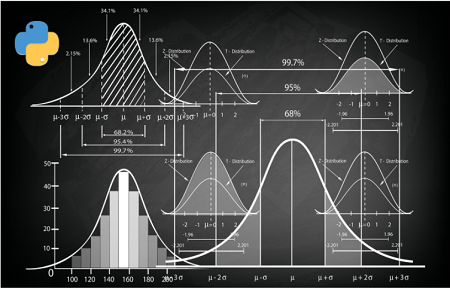## 背景知识视频教程## 了解概率密度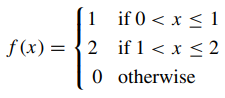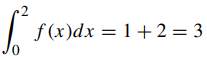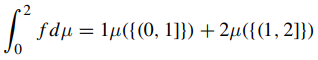## 随机变量

Ω = {1, 2, 3, 4, 5, 6}

P({1, 2, 3}) = 1/2

P({4, 5, 6}) = 1/2

P({1}) = 1/2

P({2}) = 1/2

P({3, 4, 5, 6}) = 0

Ω = {(1, 1), (1, 2), . . . , (5, 6), (6, 6)}

P((1, 2)) = P({1})P({2}) =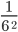dinv[(1, 6), (2, 5), (3, 4), (4, 3), (5, 2), (6, 1)]









P({1}) = P({2}) = P({3}) =P({4}) = P({5}) = P({6}) ={(1, 6), (2, 5), (3, 4), (4, 3), (5, 2), (6, 1)}

P((1, 6)) = P(1)P(6) =P((2, 5)) = P(2)P(5) =## 连续随机变量

Y :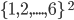→ {2, 3,..., 12}: {2, 3,..., 12} → [0, 1]

Y : A → B: B → [0, 1]

X : R → R: R → [0, 1]

X : [0, 1] → [0, 1]: [0, 1] → [0, 1]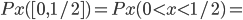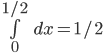=Y = 2X

Y : [0, 1] → [0, 2]Y =Y : [−1/2, 1/2] → [0, 1/4]